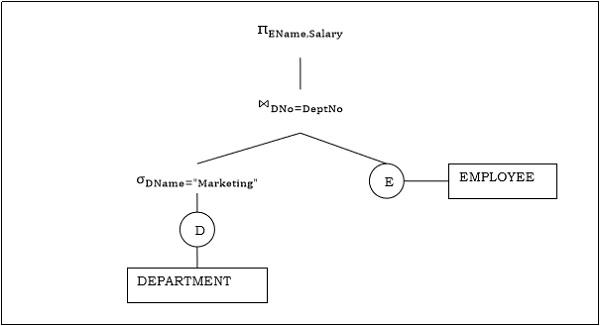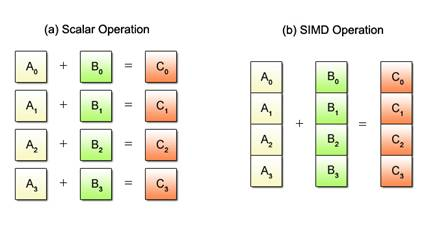tech ramblings

# Vectorization in OLAP Databases

A recent trend in OLAP (Online Analytical Processing) database systems has been to overhaul the SQL query engine from the traditional ones setup for OLTP (Online Transactional Processing) usecases. They have done this by either using vectorization, or just-in-time (JIT) compilation. In this article, I want to dive a little deep into why and how vectorization helps.

## Background

Before we go into vectorization, it would help to understand how database query engines are typically implemented. Andy Pavlo does a brilliant job at explaining it here, but to summarize, they follow what is called a "pipeline" model. Each operator in the query tree essentially calls next on its child node, which in turn calls next on its child, processes it, and returns it. And so, on ...In the image above, for example, the SELECT operator for Dname=marketing (not the same as SELECT in SQL, mind you!) calls "next" on its child, the department table scan operator. That operator scans the table, maybe sequentially or using an index, and then returns a single row back. The SELECT operator then proceeds to apply the filter and then if there is a match, returns the tuple further up the tree. In this way, each tuple is fed from the leaf nodes up to the top of the tree in a "pipeline". It's worth noting that unlike the SELECT operator which essentially processes one tuple at a time in the pipeline, for some operators, like the join for example, all the rows from one of its child would need to be materialized(i.e. collected and saved in memory) before it can proceed. These are often referred to as "pipeline-breakers".

## Vectorization

Vectorization is an implementation model that is very similar to the pipeline model, with one important difference in that each operator returns a batch of tuples as opposed to a single tuple. This is a popular technique that has been used in many popular OLAP engines like Presto, Redshift, and Snowflake. At first, it seems like a rather simple change, but it has significant performance implications.

### Reduced Invocations

A typical example of an OLAP query may be to analyze large amounts of data, and produce aggregations like sums or averages. While we take function calls for granted, the time to handle an invocation for each row, for each operator, for billions of rows, can add up pretty quickly. Vectorization means less number of invocations because of batching, meaning better performance overall.

### SIMD

Single Instruction Multiple Data (SIMD) is well, the real reason why vectorization helps. Modern processors allow you to perform the same CPU operation on multiple data with the help of 128-512 bit sized registers, in a single instruction. So, if you want to add two integer vectors of size 1000 (like in the image below), traditionally, you'd need 1000 ADD instructions. With an 8-lane SIMD, i.e. a 256-bit register to store 8 ints (with each int = 32 bits), you'd need roughly only about 1000/8=125 operations. That's a huge improvement! (theoretically, at least)In C++, you can use intrinsics which provide a way to leverage these registers on Intel hardware. These functions can be hard to grok (very much in-line with the readability struggle in fast code). But, as a small example, the function `_mm256_add_epi32(a, b)` basically adds two vectors a and b of 256 bits with 8 32-bit integers packed together!

## Example

Now, let's walk through an actual example. Let's say you want to compute the average of 100 million integers (cause, why not).

The basic pipeline model would involve two operators, the sequential scan operator, that reads each int one at a time, and an aggregation operator, that adds up all the integers and then computes the average. Pretty simple, here's some pseudo-code. The AggregationOperator has the SequentialScanOperator as its child, which it calls iteratively, until completion.

```class SequentialScanOperator:
def next():
x = #read int from memory/disk
return tuple(x,)

class AggregationOperator:
def next():
while True:
input = childOperator.next()
if not input:
break
total_sum += input
total_len += 1
return tuple(total_sum/total_len,)
```

On an Intel Xeon CPU (2.40 Ghz), this took 0.463 seconds for 100 million integers.

Now, for the vectorized model, the operators and the overall setup remains the same, except for the fact that now, each operator's next() method returns a batch of tuples instead of a single tuple. The machine that was used for the experiment had AVX2 intrinsics supported (256-bit registers essentially), so we can pack 8 integers into a register. Therefore, let's say that each operator now processes in a batch of 8 tuples. The SequentialScanOperator will now return 8 tuples, and the AggregationOperator will now read 8 input tuples from its child at a time. Of course, since we want to aggregate all the values down to a single value, the output of the AggregationOperator will be a batch, but only of 1 tuple. Here's some pseudo-code (with intrinsics):

```class SequentialScanOperator:
def next():
xv = []
for i in 1..8:
xv[i] = #read int from memory/disk
return xv

class AggregationOperator:
def next():
# initialize a 256-bit vector with 0s
__m256i aggVector = _mm256_setzero_si256();
while True:
input_tuple_vector = childOperator.next()
if not input_tuple_vector:
break
# load inputs into a 256-bit temp vector
aggVectorTemp = _mm256_set_epi32(input_tuple_vector, input_tuple_vector,
input_tuple_vector, input_tuple_vector,
input_tuple_vector, input_tuple_vector,
input_tuple_vector, input_tuple_vector);
# vectorized add temp vector to result vector
aggVector = _mm256_add_epi32(aggVector, aggVectorTemp);
total_len += 8

output = []
# load it back to a (c++) array
_mm256_store_si256((__m256i*)output, aggVector);
# final reduce of vector to a single int
total_sum = sum(output)
return [(total_sum/total_len),]
```

On the same machine, this code took 0.199 seconds. That's a 2.32x speedup! While that's great, a question that immediately comes to mind is - shouldn't we expect a speedup closer to 8, since we packed 8 integers together, and essentially performed a single instruction on them? And the answer is - not necessarily. In this example, the program is bound by memory, so the memory bandwidth usually becomes the bottleneck. With vectorization, we've sped up the computation, but we still have to read the data from memory (in the example above), or from disk. And memory is typically much slower compared to the CPU. Therefore, we don't see the gains we might expect. This SO answer explains it further!

P.S. The actual code that ran this example can be found here!